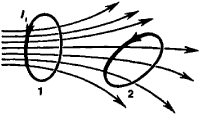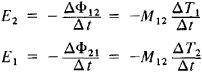# Mutual Inductance

(redirected from L12)
Also found in: Dictionary, Thesaurus, Wikipedia.

## mutual inductance

[′myü·chə·wəl in′dək·təns]
(electromagnetism)
Property of two neighboring circuits, equal to the ratio of the electromotive force induced in one circuit to the rate of change of current in the other circuit.
McGraw-Hill Dictionary of Scientific & Technical Terms, 6E, Copyright © 2003 by The McGraw-Hill Companies, Inc.
The following article is from The Great Soviet Encyclopedia (1979). It might be outdated or ideologically biased.

## Mutual Inductance

a quantity that characterizes the magnetic coupling between two or more electric circuits. If there are two conducting circuits, (1) and (2), some of the lines of magnetic induction generated by the current flowing through the first circuit will penetrate the area within the second circuit (see Figure 1)—that is, they will be coupled with the second circuit. The magnetic flux Φ12 through circuit (2) generated by the current I in circuit (1) is directly proportional to the current:

Φ12 = M12I1

The proportionality coefficient M2 depends on the dimensions and form of circuits (1) and (2), on the distance between the circuits, on their relative positions, and on the magnetic permeability of the surrounding medium. This coefficient is called mutual inductance or the coefficient of mutual induction. In the SI system of units, mutual inductance is measured in henrys.Figure 1

If current I2 flows through circuit (2), the magnetic flux Φ12 through the area of circuit (1) is also proportional to the current:

Φ21 = M21I2

where M21 = M12.

The existence of a magnetic coupling between the circuits is manifested by the fact that, upon a change of current in one circuit, an induced electromotive force appears in the adjacent circuit. According to the law of electromagnetic induction,where E2 and E1 are induced electromotive forces arising in circuits (2) and (1) and ΔΦ]2 and ΔΦ21 are the change in magnetic fluxes through the respective circuits over time Δt.

The mutual energy W12 of the magnetic field is expressed with the aid of mutual inductance for the currents I1 and I2 as follows:

Wl2 = ±M12I1I2